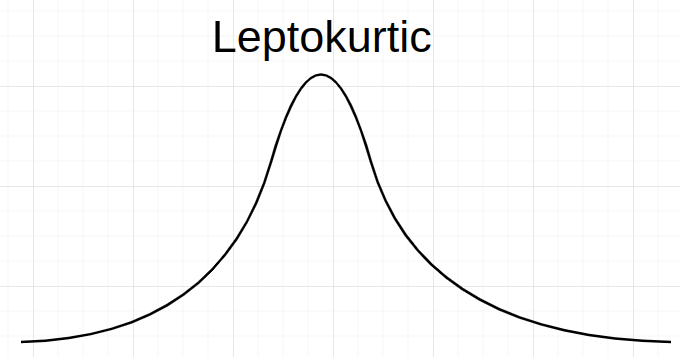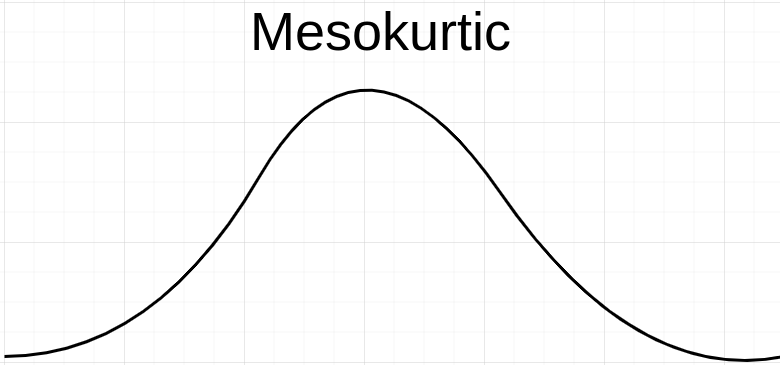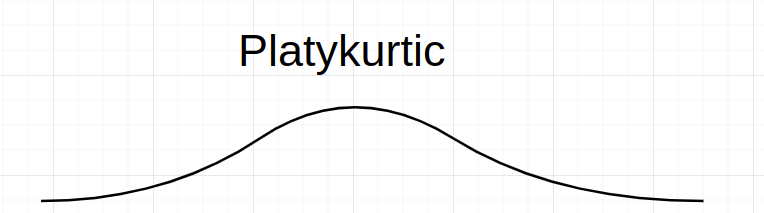# Kurtosis

Or input your data as csv

Sharing helps us build more free tools

Quickly calculate kurtosis with this free online kurtosis calculator.

Kurtosis measures how much data is in the tails of a distribution.

A distribution with large kurtosis will have "heavy tails", which means that there are a lot of outliers. A distribution with low Kurtosis is considered more "central", and will have fewer outliers.

## When To Use Kurtosis

Kurtosis is useful to measure when you want to gauge how many outliers a distribution might have. It can be used with a histogram to visualize the distribution. Skewness can be used to measure the symmetry of the distribution. A boxplot can be used to visualize the distribution's five-number summary.

## How To Calculate Kurtosis

Click the dataset input at the top of the page. Or drag and drop your dataset into the input box.

2. ### View the kurtosis of each column

A table with the kurtosis of each column will appear on the screen

## Kurtosis Formula

Kurtosis = sum(x_i - x_mean)^4 / (n * standard_deviation^4)

Where x_i is a point, x_mean is the mean of all points x, n is the number of points and standard_deviation is the standard deviation of x.

## Types of Kurtosis

The kurtosis of a set of numbers can be classified based on how it compares to the Kurtosis of the normal distribution. The normal distribution has a kurtosis of 3.

Leptokurtic distributions have a higher kurtosis than the normal distribution. These distributions have long, heavy tails. Sometimes Leptokurtic distributions are said to have positive, excess kurtosis.Mesokurtic distributions have a kurtosis of 3.Platykurtic datasets have a kurtosis of less than 3.## Excess Kurtosis

Excess kurtosis is used to compare the kurtosis of a distribution with the normal distribution.

Excess kurtosis = kurtosis - 3

Where kurtosis is the kurtosis of your dataset.

Leptokurtic distributions have a positive excess kurtosis, while platykurtic distributions have a negative excess kurtosis.

You can calculate excess kurtosis by using the kurtosis calculator and subtracting 3 from the result.